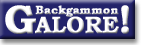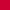TheoryForum Archive : Theory

 Optimal strategy?

 From: Gary Wong Address: gary@cs.arizona.edu Date: 2 July 1998 Subject: Re: Existence of best strategy Forum: rec.games.backgammon Google: wtd8bnu6us.fsf@brigantine.CS.Arizona.EDU

```BOSCH Holger writes:
> I have seen the posting about the "future of backgammon", and I have a
> related and maybe more fundamental question: I wonder if there exists
> a best strategy in Backgammon, i.e. a strategy that is better than any
> other strategy.

Subject to one or two restrictions, the answer is yes.  (Several strategies
that are no worse than any other strategy, at least.)

> I do not talk about roll-outs or calculations that suppose that both
> players play always the "best" move, since this has to be proven
> first. Is it theoretical impossible that there are for instance three
> strategies A, B and C, such that A is better than B, B better than C
> and C better than A (and which are better than any other strategy)?

Three strategies certainly exist such that each is better than one of the
others and worse than the third (ie. those three strategies are
non-transitive); here's one example of strategies A, B and C:

A: Form a forward anchor and make low points in home board to blitz.
B: Overly `pure' play: Slot like crazy, try to prime.  Bruce Becker :-)
C: Play safe and don't leave blots.

If you adjust the overall strengths of three players using these strategies
so they end up roughly equal in "skill", then A will tend to beat B because
his forward anchor is an excellent defence against being primed.  B will
beat C a little more often than not, because C's back men will easily be
trapped.  And it's conceivable that there is a player C who, despite losing
to B because of his weakness against primes, can nevertheless beat A
through superior skill in other areas and the fact that C will not easily
be blitzed. So these strategies are non-transitive.

However, it is _impossible_ for any set of non-transitive strategies to be
better than every remaining strategy.  The complete proof is beyond the
scope of this article (that phrase is so much more appealing than "I'm
kind of hazy on the details" ;-) but there is a theorem (the Minimax
Theorem) that states that "every finite, zero-sum, two-person game has
optimal mixed strategies" (see the definition of "Minimax theorem" in
the Encyclopaedia of Mathematics at
http://www.astro.virginia.edu/~eww6n/math/).  Money backgammon is actually
_not_ a finite game because the cube can take on arbitrarily many values,
but match backgammon is finite.

A _mixed strategy_ is a general class of strategy including those where a
player makes decisions probabilistically (an example of why this may be
necessary is the game "paper, scissors, rock" where the best strategy is to
pick a move at random -- always picking "paper" is not the best strategy).
Since backgammon is a game of complete information (both players can see
the state of the board and dice) we can actually go slightly stronger than
the Minimax theorem and claim that backgammon has optimal _pure_ strategies
(ie. strategies where the same move is always made in the same position).
Note that non-transitive strategies (eg. the A, B and C above) _cannot_ be
optimal; the Minimax theorem states that any optimal strategy must lie at a
"saddle point" of the payoff matrix.  A strategy belonging to a
non-transitive set cannot possibly lie at a saddle point because by
definition there is another strategy along the same row/column of the
matrix with a better payoff.  The practical upshot is that if you believe
the Minimax Theorem (and you can read a proof elsewhere :-) then yes, there
are optimal strategies for backgammon.  (There is more than one optimal
strategy because different actions may result in identical payoff; eg.
accepting or declining a double that would result in a dead cube when you
have exactly 25% chance of winning).

If you're a glutton for punishment and want to read about why this proof
does not suffice for money backgammon (with an unlimited cube) and an
attempt to find an alternate proof/disproof that optimal strategies exist
for money backgammon, look at http://www.dejanews.com/ for a position
posted by Paul Tanenbaum entitled "Extremely theoretical" and a discussion
with David Ullrich and others "Under-doubling dice".  We never quite got a
rigourous proof, but personally I suspect that there is an `optimal'
strategy for money backgammon too (depending how you define `optimal').

> Maybe it's trivial but I couldn't figure it out. I think, the problem
> is that in contact situations, the equities of two positions can
> depend on each other, so you can not just work backwards and calculate
> the equities. You do not even know, if and how two positions depend on
> each other, since you need to know your "best" move to do so. However,
> I might be wrong and following is correct.
>
> Gary Wong proposed the following approach (in Re: The future of
> backgammon):
> > Infinite sequences aren't a problem -- there are a finite number of
> > possible board states in backgammon, and so you can treat backgammon
> > as a Markov process over a finite number of states.  The probabilities
> > of a transition from one state to any other form an n x n matrix --
> > there IS enough information in the matrix to determine the probability
> > of reaching any terminal state (if you ignore the cube, there are 6
> > terminal states: win, gammon, and backgammon for each player); it's
> > analogous to a simultaneous equation with n equations and n unknowns.
>
> I do not know the framework of simultaneous equations, but how can you
> know that there is enough information in the matrix? If I understand
> you correctly, the matrix elements are the transition probablities
> between two states. But these probablities are also unknown (at least
> for
> some positions, i.e. most/several contact positions), since you need
> to know the best moves to compute them.

You are quite right, my explanation glossed over part of the problem.  The
statement about the Markov process and probability matrix holds if an
optimal strategy is known, but is not a practical way to find one.
Nevertheless I claim it is possible to find such a strategy by computer
(eg. the truly ugly brute force method of enumerating all strategies and
finding a saddle point of the payoff matrix).

> So, is there the Holy Grail of a best strategy out there???

Yes -- as it is written in the Gospel according to Saint John :-)
(John von Neumann proved the Minimax Theorem in 1928).

Cheers,
Gary.
--
Gary Wong, Department of Computer Science, University of Arizona
gary@cs.arizona.edu     http://www.cs.arizona.edu/~gary/
```

### Theory

Derivation of drop points  (Michael J. Zehr, Apr 1998)
Double/take/drop rates  (Gary Wong, June 1999)
Drop rate on initial doubles  (Gary Wong, July 1998)
Error rate--Why count forced moves?  (Ian Shaw+, Apr 2009)
Error rates--Repeated ND errors  (Joe Russell+, July 2009)
Inconsistencies in how EMG equity is calculated  (Jeremy Bagai, Nov 2007)Janowski's formulas  (Joern Thyssen+, Aug 2000)
Janowski's formulas  (Stig Eide, Sept 1999)
Jump Model for money game cube decisions  (Mark Higgins+, Mar 2012)
Number of distinct positions  (Walter Trice, June 1997)
Number of no-contact positions  (Darse Billings+, Mar 2004)
Optimal strategy?  (Gary Wong, July 1998)
Proof that backgammon terminates  (Robert Koca+, May 1994)Solvability of backgammon  (Gary Wong, June 1998)
Undefined equity  (Paul Tanenbaum+, Aug 1997)Under-doubling dice  (Bill Taylor, Dec 1997)Variance reduction  (Oliver Riordan, July 2003)From GammOnLineLong messageRecommended readingRecent addition

 Book Suggestions Books Cheating Chouettes Computer Dice Cube Handling Cube Handling in Races Equipment Etiquette Extreme Gammon Fun and frustration GNU Backgammon History Jellyfish Learning Luck versus Skill Magazines & E-zines Match Archives Match Equities Match Play Match Play at 2-away/2-away Miscellaneous Opening Rolls Pip Counting Play Sites Probability and Statistics Programming Propositions Puzzles Ratings Rollouts Rules Rulings Snowie Software Source Code Strategy--Backgames Strategy--Bearing Off Strategy--Checker play Terminology Theory Tournaments Uncategorized Variations